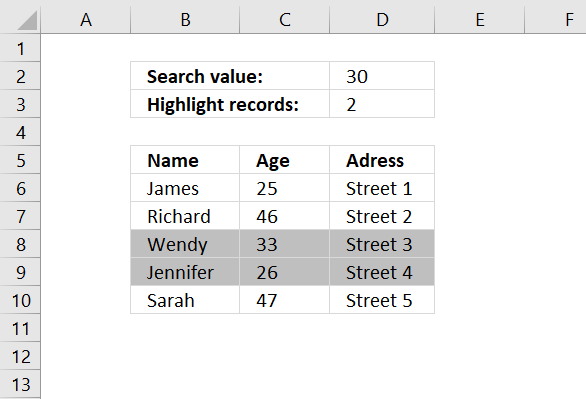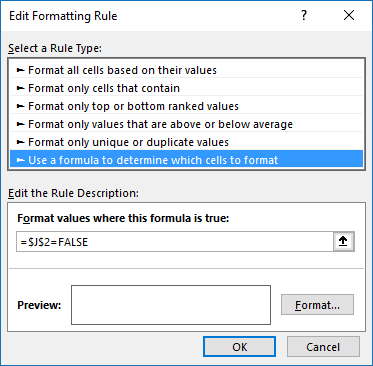Author: Oscar Cronquist Article last updated on January 11, 2019

###This post demonstrates how to highlight records with the closest value to a criterion, you can also choose to highlight the second closest value (or more).

You can also extract the number using the formula described in this article: Find closest value, perhaps you are also interested in how to find the numbers in a total closest to a given sum.

### Conditional formatting formula

=OR(ABS(\$C6-\$C\$2)=SMALL(ABS(\$C\$6:\$C\$10-\$C\$2), ROW(\$A\$1:INDEX(\$A:\$A, \$C\$3))))

This formula won't work in Excel 2010 and later Excel versions, simply create a named range containing the formula above. Then use that name as the conditional formatting formula, download the file later in this article if you need to see exactly how I did it.

### How to apply conditional formatting formula

1. Select cells B6:D10
2. Click "Home" tab
3. Click "Conditional Formatting" button
4. Click "New Rule.."
5. Click "Use a formula to determine which cells to format"
6. Type =OR(ABS(\$C6-\$C\$2)=SMALL(ABS(\$C\$6:\$C\$10-\$C\$2), ROW(\$A\$1:INDEX(\$A:\$A, \$C\$3)))) in "Format values where this formula is TRUE" window.(The formula displayed in the image above is not used in this article)
7. Click "Format.." button
8. Click "Fill" tab
9. Select a color for highlighting cells.
10. Click "Ok"
11. Click "Ok"
12. Click "Ok"

### How the conditional formatting formula works in cell C6

=OR(ABS(\$C6-\$C\$2)=SMALL(ABS(\$C\$6:\$C\$10-\$C\$2), ROW(\$A\$1:INDEX(\$A:\$A, \$C\$3))))

Step 1 - Calculate age differences

=OR(ABS(\$C6-\$C\$2)=SMALL(ABS(\$C\$6:\$C\$10-\$C\$2), ROW(\$A\$1:INDEX(\$A:\$A, \$C\$3))))

ABS(number) returns the absolute value of a number, a number without its sign.

ABS(\$C\$6:\$C\$10-\$C\$2)

becomes

ABS({25;46;33;26;47}-30)

becomes

ABS({-5;16;3;-4;17})

and returns {5;16;3;4;17}

Step 2 - Create an array with the same size as chosen records to highlight in cell C3.

=OR(ABS(\$C6-\$C\$2)=SMALL(ABS(\$C\$6:\$C\$10-\$C\$2),ROW(\$A\$1:INDEX(\$A:\$A,\$C\$3))))

ROW(\$A\$1:INDEX(\$A:\$A,\$C\$3)))

becomes

ROW(\$A\$1:INDEX(\$A:\$A,2)))

becomes

ROW(\$A\$1:\$A\$2)

and returns {1;2}

Step 3 - Return the two smallest numbers in array

=OR(ABS(\$C6-\$C\$2)=SMALL(ABS(\$C\$6:\$C\$10-\$C\$2),ROW(\$A\$1:INDEX(\$A:\$A,\$C\$3))))

SMALL(array,k) returns the k-th smallest number in this data set.

SMALL(ABS(\$C\$6:\$C\$10-\$C\$2),ROW(\$A\$1:INDEX(\$A:\$A,\$C\$3)))

becomes

SMALL({5;16;3;4;17};{1;2})

and returns {3;4}

Step 4 - Is cell value in C6 equal to any number in array?

=OR(ABS(\$C6-\$C\$2)=SMALL(ABS(\$C\$6:\$C\$10-\$C\$2),ROW(\$A\$1:INDEX(\$A:\$A,\$C\$3))))

becomes

OR(logical1, logical2, ...) checks whether any of the arguments are TRUE, and returns TRUE or FALSE. Returns FALSE if only all arguments are FALSE.

\$C6 is a relative AND absolute cell reference.

=OR(ABS(\$C6-\$C\$2)={3;4})

becomes

=OR(ABS(25-30)={3;4})

becomes

=OR(ABS(-5)={3;4})

becomes

=OR(5={3;4})

becomes

=OR({5=3,5=4})

becomes

=OR({FALSE,FALSE})

and returns FALSE. Row 6 is not highlighted.